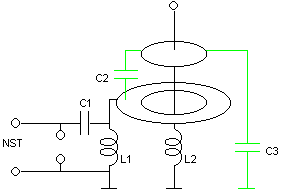A Capacitive Transformer Tesla coil

(or a 4th-order capacitively coupled multiple resonance network with distributed capacitances)This experimental device is a 4th-order multiple resonance network where the floating elements are capacitors and all the inductors are grounded. It can be interpreted as a kind of Tesla coil where the inductive transformer is replaced by a "capacitive transformer". Its schematic diagram is as shown below.C1 is charged to VC1 and the switch is closed. During the resulting transient, all the energy that was in C1 is transferred to C3+C2. C3 would be not necessary for the operation of the circuit, but its presence is unavoidable and it must be taken into account. In the actual construction, C3 and C2 are distributed capacitances. C2 is the capacitance between an interrupted metal ring connected to the lumped L1 and C1, placed around the inductor L2, and the combination of L2 and a terminal mounted above it. C3 is the remaining of thecapacitance of the terminal and of L2, coupled to the ground. The elements used are the same of my "transformerless Tesla coil". The connections are as in the figure at the left. The antenna at the top of the terminal serves as breakout point and for tuning. Another view, in this case with C1 grounded and L1 suspended (a more dangerous connection, because the NST becomes connected to the influence ring), that works practically in the same way. Note the interruption in the ring, to avoid the formation of a shorted turn around L2. (doesn't make difference, actually). The "influence ring" was made with a strip of strong aluminum foil glued to a wooden base.

The device produces corona at the teminal and arcs to insulated or grounded metal rods placed near the terminal. It also lights fluorescent lamps without contact easily. The output power is smaller than in the directly coupled system, but not much. A low-power test shows that when optimally tuned the system operates in the mode 20:21 (voltage over L1), A model corresponding to this is:

C3 = 6.05 pF
C2 = 5.18 pF
L2 = 28.2 mH
C1 = 1 nF
L1 = 315 µH

The maximum voltage gain would be 9.44. It oscillates at 276 and 290 kHz and shows complete energy transfer in 10.5 cycles (36.2 µs) of the voltage in C1. C3 is 54% of the load capacitance. The directly coupled system has a maximum gain of 9.53, but it operates in mode 9:10, with about twice faster energy transfer, having so better efficiency because with less cycles less energy is lost before being transferred.

Observe that the "capacitive transformer" in this circuit is not only capacitive. Its upper branch is formed by C2, but its lower branch is formed by C1 in parallel with L1. The "turns ratio" of this transformer is then:

n = 1+C1/C2-1/(w2C2L1)

Evaluated for w = 2p276x103 and w = 2p290x103 rads/s, the frequencies where the whole circuit resonates, the result is n = –9.46 and n = 9.46 respectively (with exact values used in the calculations), exactly the actual voltage gain that is obtained considering initial charge in C1+C2 and final energy in C2+C3, that is ((C1+C2)/(C2+C3))1/2 = 9.46 (The circuit shown above charges only C1 initially. It would charge C1 and C2 if C1 and L1 are interchanged, but the difference is insignificant). Note that as the circuit does not operate in sinusoidal steady state, the use of the "turns ratio" to predict the gain is only an approximation, that works in this case as well as it works in a regular Tesla coil with an inductive transformer.

This circuit has the same restrictions of the directly coupled system, with the gain being proportional to the number of cycles required for complete energy transfer, but in this case the presence of C3 adds another problem, turning the number of required cycles even larger. The system can be designed by a method similar to the used for the directly coupled system, and the design can be extended to higher-order systems. The ideal tuning relation is:

L1(C1+C2) = L2(C2+C3)

The system was also run with a lower impedance primary circuit, with C1 = 5.09 nF and L1 = 60.0 µH, the same elements also used in the improved version of my directly coupled system. It can be tuned to the very slow mode 41:42, with energy transfer in 72.4 µs, 21 cycles, but produces 5 cm streamers at the antenna instead of the corona of the first version.

The mreshp program can calculate the required element values for this structure, and for many others. A simpler program is also available.

Warning:

This device is powered by a power source that has enough voltage, and specially enough current, to give a fatal shock. The NST, the terminals of C1, L1, the induction ring, and the spark gap must not be touched in any circunstance while the system is energized. L2 and the terminal are also not safe to touch. The current of the arcs is high enough to cause serious burns.

Created: 14 August 2002
Last update: 07 December 2002
Created and maintained by Antonio Carlos M. de Queiroz